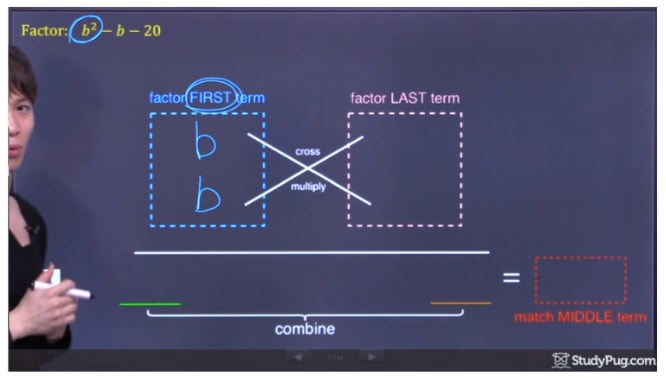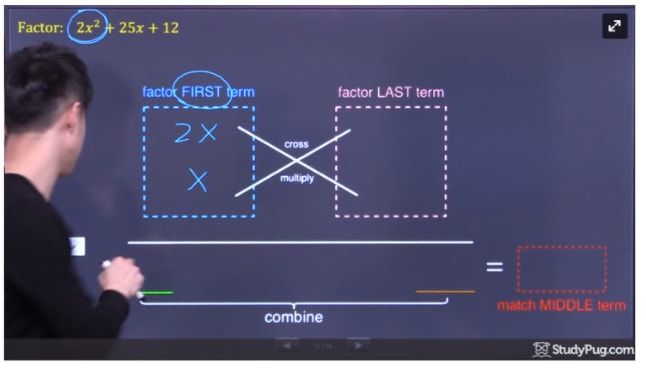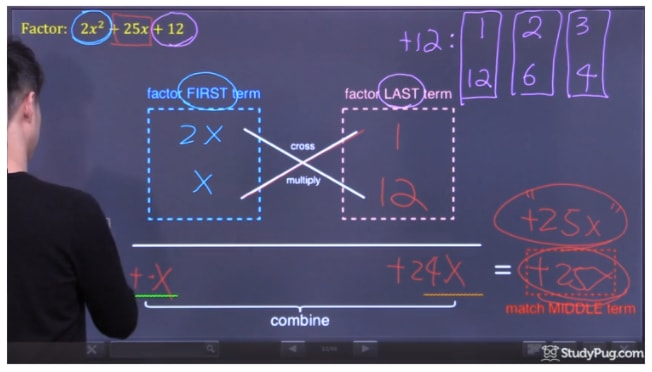# Factoring trinomials - Factoring Polynomials

## How to factor trinomials

Trinomials are algebraic expressions that has three terms in it. Quadratic trinomials are in the form of a${x^2}$ + bx + c, and the a, b, and c all stands for a number.

In order to factor trinomials, you'll have to work to find two numbers that will multiply to equal the "c" from the quadratic form above, and also add up to equal "b". These are the steps for the easier questions where the first "a" is equalled to 1. For harder problems, "a" will be a number that is not one. You'll have to first multiply "a" and "c", and then find factors of the product "a*c" that also add up to "b".

We'll explore this with example questions to demonstrate how to factor trinomials.

Question 1

Factor: ${b^2} - b - 20$

Solution:

In this example, we are going to use the "cross-multiply, then check" method to factor the trinomial. This is one of the ways to factor trinomials.

Factor the first term ${b^2}$, put the results (b and b)  in the first box.Factor the term b^2 and put them into the first box

Factor the last term -20. There are a few combinations (1x20, 2x10, 4x5) that can give us 20, so which one is it? We'll cross-multiply those combinations to the factors of the first term. See which combination will produce an answer that matches the middle term (in this question, the middle term is –b).

Out of those combinations, 4x-5 (which equals -1) is able to produce the matching middle term -b.

After all the steps, we successfully factored the trinomial  ${b^2} - b - 20$ into $\left( {b + 4} \right) \bullet \left( {b - 5} \right)$

Question 2:

Factor: $2{x^2} + 25x + 12$

Solution:

The "cross-multiply, then check" method can also be used on harder trinomials in which the leading coefficient is not 1. In this question, the leading coefficient is 2 (from the leading term $2{x^2}$).

Now, we factor the first term $2{x^2}$. The result is 2x and x. we put them in the first boxFactor the first term 2x^2 and put into the first box

In order to factor the last term +12, there are a few combinations (1x12, 2x6, 3x4). We'll once again cross-multiply those combinations to the factors of the first term. See which combination will produce a matching middle term (in this question, the middle term is +25x)

Out of those combinations, 1x12 is able to produce the matching middle term +25x. That is because (2x x 12) + (x x 1) = 24x + x = 25xFind out that 12 and 1 match the middle term of 25x

After all the steps, we successfully factored the trinomial  $2{x^2} + 25x + 12$ into $\left( {2x + 1} \right) \bullet \left( {x + 12} \right)$

## Factoring perfect square trinomials

As you do more questions that require you to do trinomial factoring, you may run into perfect square trinomials. These are trinomials that has both the "a" and "c" terms being perfect squares and the middle "b" being twice the product of the first and last term. Therefore, after factoring, you'll get an answer in the form of either (a+b)^2 or (a-b)^2.

There are good examples for you to further practice factoring trinomials. We've found some excellent online practice questions for you to try that keeps the answers hidden until you hover over the word "answer". There is also an online trinomial calculator for when you have a quadratic equation. It can help you factor any trinomial instantly! Use it to help you check your work.

### Factoring trinomials

#### Lessons

##### Factor: